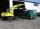# Flour

Kim needs 3/4 cup of flour to make 12 cookies. How much flour would she need to make 60 cookies?

Result

x =  3.75

#### Solution:Leave us a comment of example and its solution (i.e. if it is still somewhat unclear...):

Showing 2 comments:Nestor
This is wrong solution. The answer should be: x = 60/12 * 3/4 = 3.75Dr Math
You are right, we corrected fraction 3/5 (typo) to 3/4#### To solve this example are needed these knowledge from mathematics:

Need help calculate sum, simplify or multiply fractions? Try our fraction calculator. Do you have a linear equation or system of equations and looking for its solution? Or do you have quadratic equation?

## Next similar examples:

1. Grandmother and grandfatherGrandmother baked cakes. Grandfather ate half, then quarter of the rest ate Peter and Paul ate half of rest. For parents left 6 cakes. How many cakes maked the grandmother?
2. TeacherTeacher Rem bought 360 pieces of cupcakes for the outreach program of their school. 5/9 of the cupcakes were chocolate flavor and 1/4 wete pandan flavor and the rest were vanilla flavor. How much more chocolate flavor cupcakes than vanilla flavor?
3. Coal storageThe coal storage distribute received coal shipment within three days. The first day distribute third of the shipments, the second day of two-fifths of the rest and the third day 300 tons of coal. How many tons of coal distributed first and second day?
4. Unknown number 6Determine x if 1/6 of x is equal to 2/5 of the number 24.
5. ClassroomOne-eighth of 9th class was interested in studying at a grammar school, at a business academy one sixth, at secondary vocational schools quarter, to SOU one third and the remaining three students were interested in the school of art direction. How many st
6. EquationSolve equation and check the result: 1.4x - 3/2 + x - 9,8 = x + 0,4/3 - 7 + 1,6/6
7. Unknown number 11That number increased by three equals three times itself?
8. Unknown number 6Determine the unknown number, which is by 1.5 greater than its fourth.
9. Forest nurseryIn the forest nursery after winter, they found that 1/10 stems died out of them. For them, they land 193 new spruces. How many spruces are in the forest nursery?
10. Equation 29Solve next equation: 2 ( 2x + 3 ) = 8 ( 1 - x) -5 ( x -2 )
11. Find unknownFind unknown numerator: 4/8 + _/8 = 1
12. Simple equationSolve for x: 3(x + 2) = x - 18
13. NumberI think the number. If I add to its third seven I get same as when to its quarter add 8. Which is the number?
14. WithdrawalIf I withdrew 2/5 of my total savings and spent 7/10 of that amount. What fraction do I have in left in my savings?
15. Fraction to decimalWrite the fraction 3/22 as a decimal.
16. Mixed2improperWrite the mixed number as an improper fraction. 166 2/3
17. Passenger boatTwo-fifths of the passengers in the passenger boat were boys. 1/3 of them were girls and the rest were adult. If there were 60 passengers in the boat, how many more boys than adult were there?